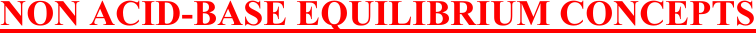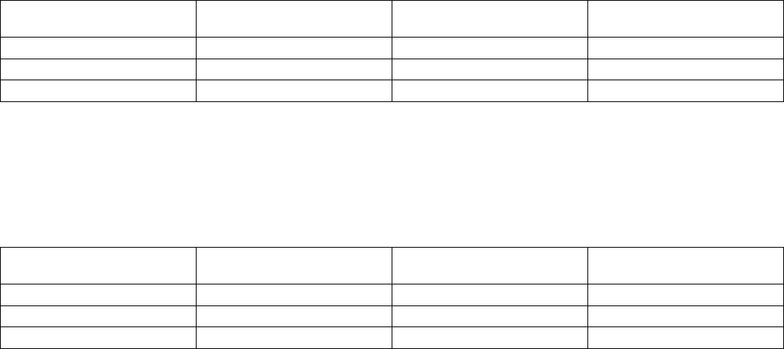Study Guides (380,000)
US (220,000)
Rutgers (3,000)
3:40 (100)
Final

01:160:161 Chapter Notes - Chapter 16: Joule, Reaction Quotient, Endothermic ProcessExam

Department
Chemistry
Course Code
01:160:161
Professor
Asbed Vassilia
Study Guide
Final

This preview shows pages 1-3. to view the full 41 pages of the document.1
CHAPTER 16 (TRO)
NON ACID/BASE CHEMICAL EQUILIBRIUM
Problems to prepare students for hourly exam II.
Non acid-base equilibrium concepts
Non acid-base equilibrium calculations
Le Chatelier’s Principle
E. Tavss 2/17

Only pages 1-3 are available for preview. Some parts have been intentionally blurred.2
NON ACID-BASE EQUILIBRIUM CONCEPTS
Chem 162-2011 Hourly Exam II + Answers
Chapter 14 - Non Acid/Base Chemical Equilibrium
Non acid-base equilibrium concepts
13. 2NO(g) + Cl2(g) 2NOCl(g) Kp =1.40 x 105 at 298K
What is Kp at 298 K for the reaction: NOCl(g) NO(g) + ½ Cl2(g)
A. 7.14 x 10-6
B. 3.57 x 10-6
C. 1.34 x 10-3
D. 5.34 x 10-3
E. 2.67 x 10-3
The equilibrium equation is reversed and halved. Therefore, the new K is the square root of the inverse of the
original K. (1/(1.40x105))0.5 = 2.67 x 10-3
Chem 162-2011 Hourly Exam II + Answers
Chapter 14 - Non Acid/Base Chemical Equilibrium
Non acid-base equilibrium concepts
10. Which of the following statements concerning the reaction quotient Q and/or the equilibrium
constant K is false?
A. Q must be equal to K at equilibrium.
B. The larger the value of K, the farther the forward reaction proceeds toward
completion.
C. As the temperature increases, the value of K increases.
D. The concentrations of pure solids and pure liquids are omitted from the
expression for Q, because they do not change during the course of a chemical
reaction.
E. By comparing the value of Q with K one can predict the direction of
change for a system not initially in equilibrium.
A. True. If Q = K, then the system is at equilibrium.

Only pages 1-3 are available for preview. Some parts have been intentionally blurred.3
B. True. The larger the value of K, the further the reaction goes to the right (which means, toward
completion).
C. False. As the temperature increases in an endothermic reaction, the value of K increases; but as the
temperature increases in an exothermic reaction the value of K decreases.
D. True. Whether the measurement is Q or K, the concentrations of pure solids and pure liquids are
omitted from the equilibrium expression because only substances that change in concentration are
included, and pure solids and pure liquids don’t change in concentration.
E. True. If Q is smaller than K then the reaction will go to the right; if Q is larger than K then the
reaction will go to the left.
CHEM 162-2010 EXAM II
Chapter 14 - Non Acid/Base Chemical Equilibrium
Non acid-base equilibrium concepts
25. SO2Cl2(g)
SO2(g) + Cl2(g) K = 0.070
Flask X: 0.50 mol SO2Cl2, 0.10 mol SO2, 0.20 mol Cl2 in 0.50L
Flask Y: 0.50 mol SO2Cl2, 0.10 mol SO2, 0.20 mol Cl2 in 1.00L
Flask Z: 0.50 mol SO2Cl2, 0.10 mol SO2, 0.20 mol Cl2 in 2.00L
Which flask will result in a reaction forming more Cl2?
D. Flasks X and Y only
E. Flasks Y and Z only
SO2Cl2(g)
SO2(g) + Cl2(g)
Initial 1.00 0.20 0.40
Change
Equilibrium
Q = ([SO2][Cl2])/[SO2Cl2]
Q = ([0.20][0.40])/[1.00] = 0.080. Q > K. Reaction will go to the left; [Cl2] will be decreased.
SO2Cl2(g)
SO2(g) + Cl2(g)
Initial 0.50 0.10 0.20
Change
Equilibrium